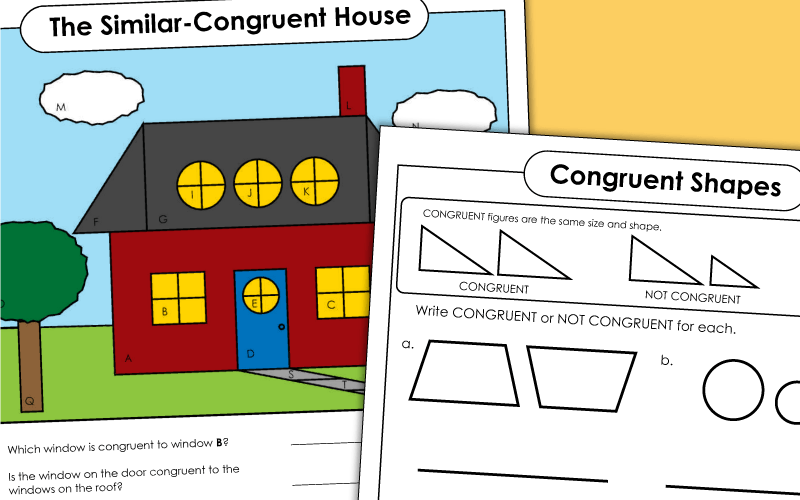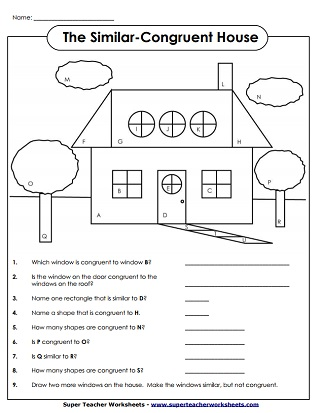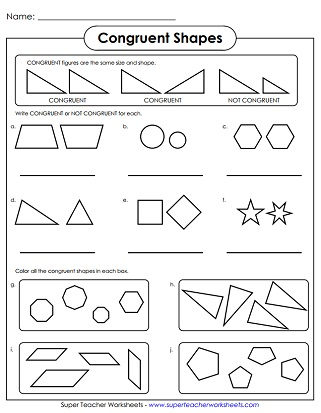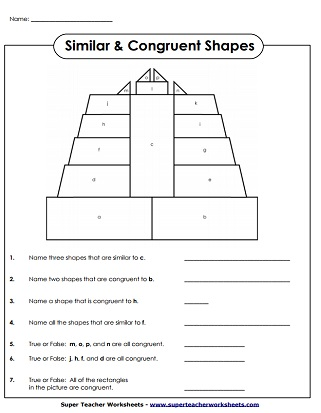# Math Geometry Worksheets

Congruent polygons are the same size and shape.  Similar polygons have the same shape, but can be different sizes.Tell whether the pairs of shapes are congruent or not congruent.  Then, color the congruent sets.
Tell whether each pair of shapes is congruent or not congruent.
Tell whether each pair of shapes is similar or not similar.  Then, color the similar shapes in each set.
Tell whether each pair of shapes is congruent or not congruent.
Tell whether each pair of shapes is congruent or not congruent.

Slide, Flip, Turn Worksheets

Flipping, Sliding, and Turning geometrical shapes

## Worksheet ImagesMy Account
Site Information